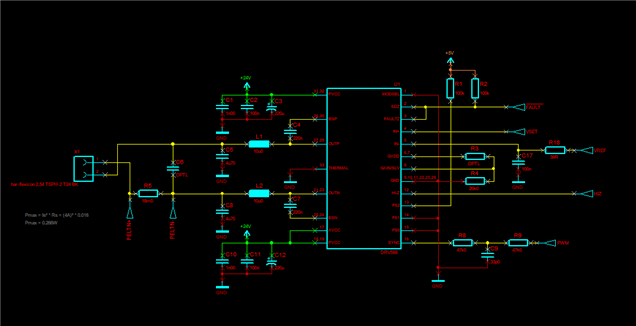If you have a related question, please click the "Ask a related question" button in the top right corner. The newly created question will be automatically linked to this question.

# DRV595: Selected doesn't match with expected gain.

Part Number: DRV595

Hello,

i was alpha testing our new hardware and realized that the selected gain wasn't matching with our expected gain at all. However, the datasheet doesn't really tell how to calculate the output values in reference to the input values at all, so I was expecting that it should be simply "G = 10 * log(Pout/Pin)" where G is 20db (Pout is the output and Pin is the input power). The datasheet tells if you select 20db gain in Master-Mode, you'll have an differential input resistance of 60kOhm (Page 10: Table 1. GAIN and MASTER/SLAVE, I assumed the differential part). Therefore the new equation is "G = 10 * log(Pout * Rin / dUin²)" (Rin --> differential input resistance, dUin --> differential input voltage).

Now I fixed the IN- Pin to 2.5V and set the IN+ Input to 2.6V. After that I could measure an output current of 0.583A and an differential output voltage of 1.049V. When I put those values in the equation above, I get around 65.65db as gain.

Have I maybe made the wrong assumptions?Regards,

Andreas

• I would like to add, that I now measured the differential input current, input voltage, output current and output voltage. The result for the input current is 1,8µA, the other values didn't change. If I calculate the gain again, I get nearly the same result of 65.312 db.

• Hi Andreas

Please use voltage to do the calculation instead of power. The input power is not all from the input pin, but will also from the power source and transferred inside of our devices, you can't get the specific data. Use  G = 20 * log(Vout / Vin) will be more easy to calculate. Use differential voltage at the both side.

• Hello,

okay, now I get it. So the unit is dbV and not db. Thank you very much.